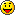# One Equals Zero

## Proving 1=0, 2=1, and so on…

Here is a pretty tricky proof that I’ve come across several times. It basically proves that a+b=a, which would mean that 1+0=0, 1=0, 2=1, and so on.

I know very well how to prove this wrong, but do you?a = b

ab = b²

ab - a² = b² - a²

a(b - a) = (b + a)(b - a)

a = b + a

If a and b are 1, then 1 = 1 + 1

1 = 2

0 = 1

Added on Sep 26, 2006 | Last Updated Sep 26, 2006

#### Responses

george says...

no

Bobby says...

im probably a little late but at the part where a(b-a)=(b+a)(b-a)

since b=a
b-a=0 and if u multiply 0 each side you would end up with 0

dave says...

your dividing by zero.  you cant divide both sides by (b-a). dividing by zero is undefined

kenneth says...

a(b - a) = (b + a)(b - a)
-(b - a)         -(b - a)
a = (b + a)

Its not equal

kenneth says...

However

"0 IS 1 number" on the number scale.
In the begining is- 0 we now have 1 number. Oh its now 2. or is it three? Now im counting 4! ah <><>
Its called Axiom of infinity i think?Enter the code from image: